Smartick is a fun way to learn math!Oct11

# Learn How to Divide with 3-Digit Numbers

Today, we’re going to learn how to divide with 3-digit numbers. Before we start, we’re going to do a quick review on the parts of a division problem. Do you remember what a dividend is? And what about the remainder? I’ve prepared a diagram for you to help jog your memory.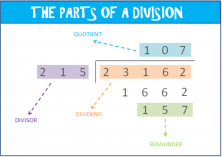Good, now we’re going to explain how to solve a 3-digit division problem in 5 simple steps.

#### Step #1. Observe how many digits the divisor has.

In our example, the divisor has 3 digits.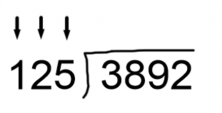#### Step #2. In the dividend, start with the same number of digits that you found in Step #1.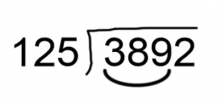#### Step #3. Compare the 3 digits from the dividend and divisor.

• If the dividend’s number is greater, we can begin dividing. In our example, we see that 389 is greater than 125, so let’s get started!
• If the dividend’s number is less than the divisor, we must use another digit from the dividend. We’ll look at an example of this situation at a different time.

#### Step #4. Divide the dividend’s numbers by the divisor’s numbers.

Divide the dividend’s first number (which is 3 in our example) by the divisor’s first number (1). 3 divided by 1 is 3. Then, we multiply our divisor (125) by 3 and see that it fits in (in other words, that it’s less than) the dividend’s 3 numbers. So, we place the result underneath the dividend’s 3 digits and subtract.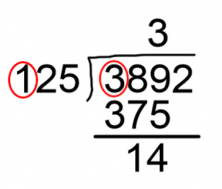#### Step #5. Bring down the dividend’s next number and divide as you did in the last step until there are no more numbers left.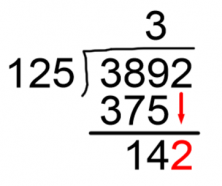After bringing down the 2, now we need to divide 142 by 125. The first digit of each number is 1, so we go with 1 and write 1 in the quotient. Then, we multiply 125 x 1 and get 125; 125 fits in 142, so we can move on to subtract it.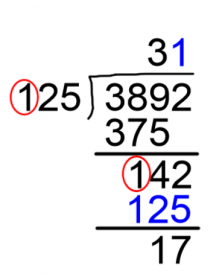Now we don’t have any more numbers left from the dividend to bring down. And just like that, we divided 3892 by 125.

The answer is 31, which is the quotient, and we have a remainder of 17.

Quickly, we’re going to check our answer. Do you know how to check a division problem?

Dividend = divisor x quotient + remainder

Let’s put it to the test. What is 125 x 31 + 17? 3892. That’s just the same value as the dividend.

Checked and successful!

Be careful! If we don’t get the same answer when you check the problem, we have to retrace our steps because it means that something went wrong in our calculations.

I hope this helped you learn how to divide with 3-digit numbers.

If you want to learn lots of math, try out Smartick free.